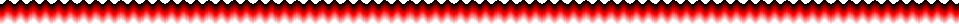The For...Next LoopIn this lesson you'll learn how the For...Next Loop works exactly, how to use it, and how it's useful.  This is the basic syntax of the For...Next Loop

 For countervariable = initialvalue To endvalue [ Step stepvalue ]    ...statements Next countervariable

First of all, we'll explain what happens in this.

1. The program reaches the For line and first sets the countervariable to whatever Integer the initialvalue is, then if  [ Step stepvalue ] is added (it's optional), it checks the value of stepvalue:
• If stepvalue is positive and initialvalue is greater then endvalue then that means that the loop has already done it's job, so it goes to the line following the Next line.
• If stepvalue is negative and initialvalue is less then endvalue then that also means that the loop has already done it's job, so it goes to the line following the Next line.
• Any other case, it continues to the next step.
2. Next (hehehaha) it checks if the value of countervariable is greater then endvalue (or less then if stepvalue is negative), if it is, then the loop is exited, all else, it gets down to the next lines
3. The Loop completes whatever statements are between for and next.
4. It reaches the "Next countervariable" line, this line sets countervariable to countervariable + stepvalue, then it sends the program back up the the For line with the same variable (Goes back to step 2).

Since that's PROBABLY pretty complicated, we'll give some examples:

 For x = 1 To 10   Me.Print X     Next x

We'll go over it just like we did with the general one above:

1. The program reaches the For line and first sets the x (countervariable) to 1 (initialvariable), then since Step and stepvalue aren't there, it assumes stepvalue is 1
• stepvalue is +1, and initialvalue is less then endvalue, so the loop still has work to do, so on to the next step.
2. Next (hehehaha) it checks if x is greater then 10 (the first time x = 1), and if it is, then it exits the loop, and goes on to the line after the "Next x" line .
3. The Loop prints the value of x on the form.
4. It reaches the "Next x" line, this line sets x to x + 1 [x + stepvalue], then it sends the program back up the the For x line (Goes back to step 2).

After this loop ends, the value of x will be 11, the loop goes through, until x = 10, then it goes through the print line, then the next line sets x to x + 1 (11), then the program processes the for line, which checks the value of x, since this time it is 11, the loop exits, and x is still 11.  Also, on the form will be the numbers 1 to 10, on individual lines (because of how Me.Print works)

That's a normal situation, now some more complicated ones:

 For x = 10 To 1 Step -1   Me.Print X      Next x

We'll go over it just like we did with the general one above:

1. The program reaches the For line and first sets the x (countervariable) to 10 (initialvariable), then remembers the stepvalue of -1
• stepvalue is -1, and initialvalue is greater then endvalue, so the loop still has work to do, so on to the next step.
2. Next (hehehaha) it checks if x is less then then 1 (the first time x = 10), and if it is, then it exits the loop, and goes on to the line after the "Next x" line .
3. The Loop prints the value of x on the form.
4. It reaches the "Next x" line, this line sets x to x - 1 [x + stepvalue], then it sends the program back up the the For x line (Goes back to step 2).

After this loop ends, the value of x will be 0, this is because the loop goes through, until x = 1, then it goes through the print line, then the next line sets x to x - 1 (0), then the program processes the for line, which checks the value of x, since this time it is 0, the loop exits, and x is still 0.  Also, on the form will be the numbers 10 to 1, on individual lines.

Simple enough?  Now some values that will skip the For...Next Loop

 1 For x = 10 To 1   Me.Print X      Next x

 2 For x = 1 To 10 Step -1   Me.Print X      Next x

A quick run through about why these loops are "ignored."

1. Since stepvalue isn't there, it is set to 1 by default, then it checks and sees that since stepvalue is positive, and initialvalue is greater then endvalue, that means that the loop has finished it jobs, so it goes to the line after Next x.
2. stepvalue is -1.  It checks and sees that since stepvalue is negative, and initialvalue is less then endvalue, that means that the loop has finished it jobs, so it goes to the line after Next x.

So that explains the For...Next Loop, hopefully explaining it better then I did during Gym for Nick lol. Any other questions Email me.

* Home * VB Programming Guides
 ~~